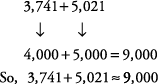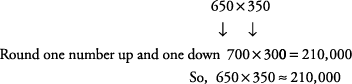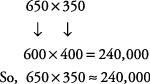## Estimating Sums, Differences, Products, and Quotients

Knowing how to approximate or estimate not only saves you time but can also help you check your answer to see whether it is reasonable.

#### Estimating sums

Use rounded numbers to estimate sums.

##### Example 1

Give an estimate for the sum 3,741 + 5,021 rounded to the nearest thousand.Note: The symbol ≈ means is approximately equal to.

#### Estimating differences

Use rounded numbers to estimate differences.

##### Example 2

Give an estimate for the difference 317,753 – 115,522 rounded to the nearest hundred thousand.#### Estimating products

Use rounded numbers to estimate products.

##### Example 3

Estimate the product of 722 × 489 by rounding to the nearest hundred.##### Example 4

Estimate the product of 650 × 350 by rounding to the nearest hundred.You can also round the first number down and the second number up and get this estimate:In either case, your approximation is closer than it will be if you round both numbers up, which is the standard rule.

#### Estimating quotients

Use rounded numbers to estimate quotients.

##### Example 5

Estimate the quotient of 891 ÷ 288 by rounding to the nearest hundred.